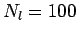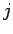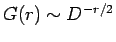Next: 3.7 Conclusion Up: 3. Many-Mody Multi-Scale Method Previous: 3.5.4 Real Space Ansatz

# 3.6 Numerical Considerations and Outlook

In the development of this MSMB method we were forced to employ various approximations due to current computational limitations. The largest numerical concession was made in section 3.3 where we restricted the calculations ofto the small cluster (see Eq. 3.14). Ideally, the irreducible verticeswould be interpolated and the full reducible vertex evaluated on the large cluster in turn. This calculation however would scale as(is the number of time-slices in the QMC(2)) and hence provide little advantage over a single cluster QMC calculation. We therefore restrict the evaluation ofin Eq. 3.18 to the small cluster and interpolate the product ofandto the large cluster.

With the onset of peta-scale computing we will be able to make two fundamental improvements to the MSMB approach in the near future. Initially, we will gain the ability to include the fully momentum and frequency dependentin our calculation, thus eliminating the necessity of the-approximation (Eq. 3.9). Inherent in this modification is an explicit account of the correct short ranged physics hence removing the need of the Ansatz. However, the memory and CPU requirements for this type of calculation scale as. For a large cluster withand, this would require 66G of double precision complex storage, far exceeding the memory associated with a single CPU. These staggering memory requirements can currently only be met by some shared-memory parallel processing (SMP) super-computers.

In the second improvement, the approximation of the large clusterby the small cluster QMC one can be replaced by one utilizing the fully irreducible vertex(the vertex which is two-particle irreducible in both the horizontal and vertical plane). This results in a self-consistent renormalization ofvia the Parquet equations (16,23), and hence an inclusion of long ranged correlations in the crossing channel (see Fig. 3.15) which are missing in both the-approximation and the-based approximation described above.The superiority of the latter approach becomes clear in the high-dimensional limit, where it is, not, which becomes local. This can be shown by considering the simplest non-local corrections to the respective verticesandin Fig. 3.16. The boxes represent a set of graphs restricted to site(local) and(neighboring) respectively. In the limit of high dimensions, each sitehasadjacent sites. The contributions of each leg within the vertex in the limitis(for details see Ref. (8)). This results in a contribution to the correction offor the two legs inandfor. Thus, the non-local corrections toincluding all neighboring sitesfalls off asand becomes local in the infinite-dimensional limit. In contrast, the corrections toremain of order one. Therefore, in the high-dimensional limit,is local whilehas non-local corrections. In finite dimensions, we would expect thatis more compact thanwhenever the single-particle Green function falls quickly with distance. Thenshould always be better approximated by a small cluster calculation than. (Despite the fact thathas non-local corrections, one can easily show that in the high-dimensional limit, all of the methods discussed here will yield the same self energy and susceptibilities since the non-local corrections tofall on a set of zero measure points).

In employing the solution to the Parquet equation in a MSMB method we would be able to resolve two major limitations of the current approach: 1) An implementation considering the full frequency and momentum dependent vertex will be devoid of the causality problems associated with the self-energy mixing of the two cluster sizes. 2) The approach constitutes a conserving approximation for the large cluster self-energy. Given these potential gains of a future method, we have to stress the extensive computational demands associated with this approach. While in abased implementation a trivial numerical parallelization of the problem leaves manageable demands, the complex nature of the Parquet approach requires substantial future development.Next: 3.7 Conclusion Up: 3. Many-Mody Multi-Scale Method Previous: 3.5.4 Real Space Ansatz
© Cyrill Slezak